# subplot

## 语法

``subplot(m,n,p)``
``subplot(m,n,p,'replace')``
``subplot(m,n,p,'align') ``
``subplot(m,n,p,ax)``
``subplot('Position',pos)``
``subplot(___,Name,Value)``
``ax = subplot(___)``
``subplot(ax)``

## 说明

``subplot(m,n,p)` 将当前图窗划分为 `m`×`n` 网格，并在 `p` 指定的位置创建坐标区。MATLAB® 按行号对子图位置进行编号。第一个子图是第一行的第一列，第二个子图是第一行的第二列，依此类推。如果指定的位置已存在坐标区，则此命令会将该坐标区设为当前坐标区。`

``subplot(m,n,p,'replace')` 删除位置 `p` 处的现有坐标区并创建新坐标区。`
``subplot(m,n,p,'align') ` 创建新坐标区，以便对齐图框。此选项为默认行为。`

``subplot(m,n,p,ax)` 将现有坐标区 `ax` 转换为同一图窗中的子图。`

``subplot('Position',pos)` 在 `pos` 指定的自定义位置创建坐标区。使用此选项可定位未与网格位置对齐的子图。指定 `pos` 作为 `[left bottom width height]` 形式的四元素向量。如果新坐标区与现有坐标区重叠，新坐标区将替换现有坐标区。`
``subplot(___,Name,Value)` 使用一个或多个名称-值对组参数修改坐标区属性。在所有其他输入参数之后设置坐标区属性。`

``ax = subplot(___)` 创建一个 `Axes` 对象、`PolarAxes` 对象或 `GeographicAxes` 对象。以后可以使用 `ax` 修改坐标区。`

``subplot(ax)` 将 `ax` 指定的坐标区设为父图窗的当前坐标区。如果父图窗尚不是当前图窗，此选项不会使父图窗成为当前图窗。`

## 示例

```subplot(2,1,1); x = linspace(0,10); y1 = sin(x); plot(x,y1) subplot(2,1,2); y2 = sin(5*x); plot(x,y2)``````subplot(2,2,1) x = linspace(0,10); y1 = sin(x); plot(x,y1) title('Subplot 1: sin(x)') subplot(2,2,2) y2 = sin(2*x); plot(x,y2) title('Subplot 2: sin(2x)') subplot(2,2,3) y3 = sin(4*x); plot(x,y3) title('Subplot 3: sin(4x)') subplot(2,2,4) y4 = sin(8*x); plot(x,y4) title('Subplot 4: sin(8x)')``````subplot(2,2,1); x = linspace(-3.8,3.8); y_cos = cos(x); plot(x,y_cos); title('Subplot 1: Cosine') subplot(2,2,2); y_poly = 1 - x.^2./2 + x.^4./24; plot(x,y_poly,'g'); title('Subplot 2: Polynomial') subplot(2,2,[3,4]); plot(x,y_cos,'b',x,y_poly,'g'); title('Subplot 3 and 4: Both')``````for k = 1:4 data = rand(1,10); subplot(2,2,k) stem(data) end````subplot(2,2,2,'replace')````pos1 = [0.1 0.3 0.3 0.3]; subplot('Position',pos1) y = magic(4); plot(y) title('First Subplot') pos2 = [0.5 0.15 0.4 0.7]; subplot('Position',pos2) bar(y) title('Second Subplot')``````figure ax1 = subplot(2,1,1,polaraxes); theta = linspace(0,2*pi,50); rho = sin(theta).*cos(theta); polarplot(ax1,theta,rho) ax2 = subplot(2,1,2,polaraxes); polarscatter(ax2,theta,rho)``````ax1 = subplot(2,1,1); Z = peaks; plot(ax1,Z(1:20,:)) ax2 = subplot(2,1,2); plot(ax2,Z)```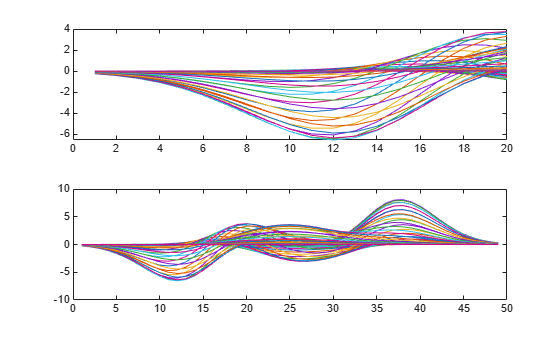```ax1.FontSize = 15; ax2.LineWidth = 2;``````for k = 1:4 ax(k) = subplot(2,2,k); end subplot(ax(2)) x = linspace(1,50); y = sin(x); plot(x,y,'Color',[0.1, 0.5, 0.1]) title('Second Subplot') axis([0 50 -1 1])``````x = linspace(1,10); y = sin(x); plot(x,y) title('Sine Plot')```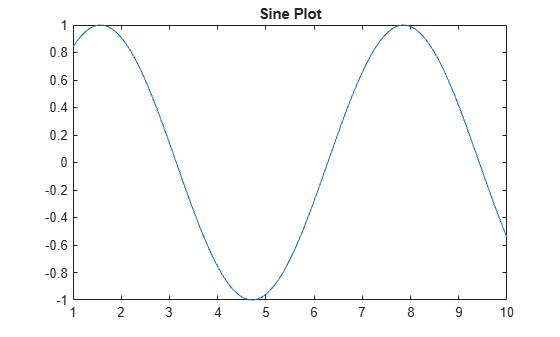```ax = gca; subplot(2,1,2,ax)``````figure x = linspace(0,10); y1 = sin(x); plot(x,y1) title('Line Plot 1') ax1 = gca;```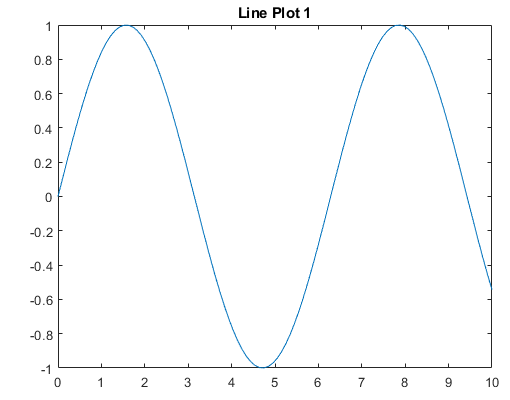```figure y2 = 2*sin(x); plot(x,y2) title('Line Plot 2') lgd = legend('2*Sin(x)'); ax2 = gca;```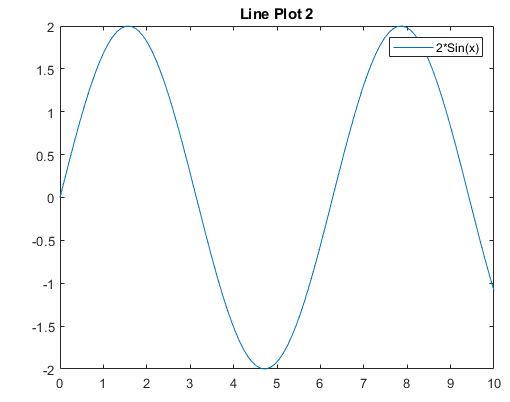```fnew = figure; ax1_copy = copyobj(ax1,fnew); subplot(2,1,1,ax1_copy) copies = copyobj([ax2,lgd],fnew); ax2_copy = copies(1); subplot(2,1,2,ax2_copy)```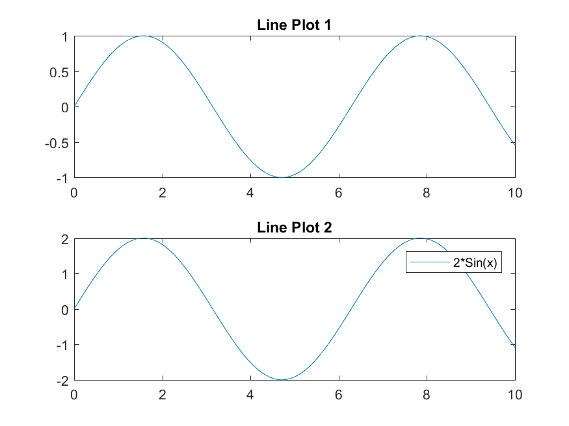## 输入参数

• 如果 `p` 是正整数标量，则 `subplot` 在网格位置 `p` 处创建一个子图。

• 如果 `p` 是正整数向量，则 `subplot` 新建一个跨 `p` 中列出的网格位置的子图。

• `left``bottom` 元素指定子图的左下角相对于图窗的左下角的位置。

• `width``height` 元素指定子图维度。

## 提示

• 要清除图窗的内容，请使用 `clf`。例如，您可以在创建新子图布局之前清除图窗中的现有子图布局。

• 要叠加坐标区，请改用 `axes` 命令。`subplot` 函数会删除与新坐标区重叠的现有坐标区。例如，`subplot('Position',[.35 .35 .3 .3])` 会删除所有底层坐标区，但 `axes('Position',[.35 .35 .3 .3])` 会将新坐标区置于图窗的中部而不删除底层坐标区。

• `subplot(111)` 是一个例外，其行为与 `subplot(1,1,1)` 不同。出于向后兼容的原因，`subplot(111)` 是子图的一种特殊情况，它不立即创建坐标区，而是设置图窗，以便接下来的图形命令执行 `clf reset`。接下来的图形命令将删除所有图窗子级，并在默认位置创建新的坐标区。`subplot(111)` 不返回 `Axes` 对象，如果代码指定了返回参数，将会发生错误。

## 替代功能

• 对绘图之间和布局边缘周围间距的控制

• 布局顶部共享标题的选项

• 共享 x 轴和 y 轴标签的选项

• 用于控制分块是固定大小还是可以调整的可变大小的选项